# Contour integral facts for kids

Kids Encyclopedia Facts

In complex analysis, contour integration is a way to calculate an integral around a contour on the complex plane. In other words, it is a way of integrating along the complex plane.

More specifically, given a complex-valued function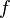$f$ and a contour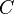$C$, the contour integral of$f$ along$C$ is written as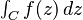$\textstyle \int_C f(z) \, dz$ or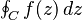$\textstyle \oint_C f(z) \, dz$.

## Calculating contour integrals with the residue theorem

For a standard contour integral, we can evaluate it by using the residue theorem. This theorem states that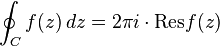$\oint_C f(z)\,dz= 2\pi i\cdot \text{Res} f(z)$

where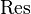$\text{Res}$ is the residue of the function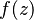$f(z)$,$C$ is the contour located on the complex plane. Here,$f(z)$ is the integrand of the function, or part of the integral to be integrated.

The following examples illustrate how contour integrals can be calculated using the residue theorem.

### Example 1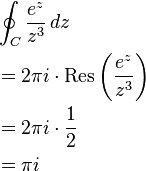\begin{align} &\oint_C \frac{e^z}{z^3}\,dz\\ &=2\pi i\cdot \text{Res} \left(\frac{e^z}{z^3}\right)\\ &=2\pi i\cdot \frac{1}{2}\\ &=\pi i \end{align}

### Example 2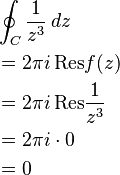\begin{align} &\oint_C \frac{1}{z^3}\,dz\\ &=2\pi i \, \text{Res} f(z)\\ &=2\pi i \, \text{Res} \frac{1}{z^3}\\ &=2\pi i\cdot 0\\ &=0 \end{align}

## Multivariable contour integrals

To solve multivariable contour integrals (contour integrals on functions of several variables), such as surface integrals, complex volume integrals and higher order integrals, we must use the divergence theorem. For right now, let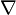$\nabla$ be interchangeable with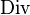$\text{Div}$. These will both serve as the divergence of the vector field written as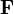$\mathbf{F}$. This theorem states that:$\underbrace{ \int \cdots \int_U }_n \text{Div}(\mathbf{F}) \, dV = \underbrace{ \oint \cdots \oint_{\partial U} }_{n-1} \mathbf{F} \cdot \mathbf{n} \, dS$

In addition, we also need to evaluate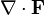$\nabla\cdot \mathbf{F}$, where$\nabla \cdot \mathbf{F}$ is an alternate notation of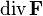$\text{div}\,\mathbf{F}$. The divergence of any dimension can be described as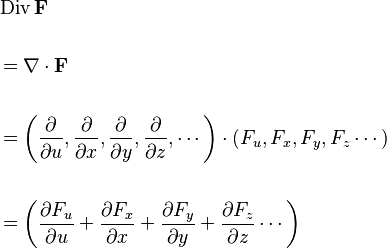\begin{align} &\operatorname{Div}{\mathbf{F}}\\\\ &=\nabla\cdot\textbf{F}\\\\ &= \left(\frac{\partial}{\partial u}, \frac{\partial}{\partial x}, \frac{\partial}{\partial y}, \frac{\partial}{\partial z},\cdots \right) \cdot (F_u,F_x,F_y,F_z\cdots)\\\\ &=\left(\frac{\partial F_u}{\partial u} + \frac{\partial F_x}{\partial x} + \frac{\partial F_y}{\partial y} + \frac{\partial F_z}{\partial z}\cdots \right) \end{align}

The following examples illustrate the use of divergence theorem in the calculation of multivariate contour integrals.

### Example 1

Let the vector field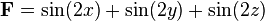$\mathbf{F}=\sin(2x)+\sin(2y)+\sin(2z)$ be bounded by the following conditions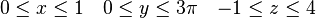${0\leq x\leq 1} \quad {0\leq y\leq 3\pi} \quad {-1\leq z\leq 4}$

The corresponding double contour integral would be set up as such: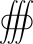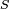${S}$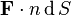${\mathbf{F} \cdot n} \, {\rm d}\,S$

We now evaluate$\nabla\cdot\mathbf{F}$ by setting up the corresponding triple integral: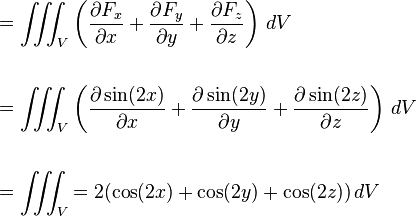\begin{align} &=\iiint_V \left(\frac{\partial F_x}{\partial x} + \frac{\partial F_y}{\partial y} + \frac{\partial F_z}{\partial z}\right)\,dV\\\\ &=\iiint_V \left(\frac{\partial \sin(2x)}{\partial x} + \frac{\partial \sin(2y)}{\partial y} + \frac{\partial \sin(2z)}{\partial z}\right)\,dV\\\\ &=\iiint_V {{= 2(\cos(2 x) + \cos(2 y) + \cos(2 z))}}\,dV \end{align}

From this, we can now evaluate the integral as follows: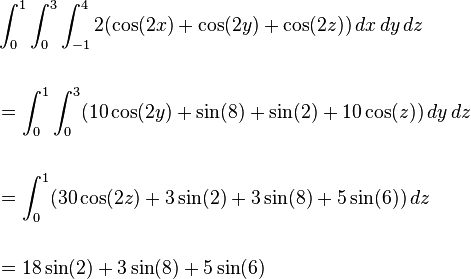\begin{align} &\int_{0}^{1}\int_{0}^{3}\int_{-1}^{4} 2(\cos(2x)+\cos(2y)+\cos(2z))\,dx\,dy\,dz\\\\ &=\int_{0}^{1}\int_{0}^{3}(10\cos(2y)+\sin(8)+\sin(2)+10\cos(z))\,dy\,dz\\\\ &=\int_{0}^{1}(30\cos(2z)+3\sin(2)+3\sin(8)+5\sin(6))\,dz\\\\ &=18\sin(2)+3\sin(8)+5\sin(6) \end{align}

### Example 2

Given the vector field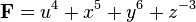$\mathbf{F}=u^4+x^5+y^6+z^{-3}$ and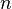$n$ being the fourth dimension. Let this vector field be bounded by the following: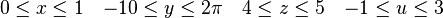${0\leq x\leq 1} \quad {-10\leq y\leq 2\pi} \quad {4\leq z\leq 5} \quad {-1\leq u\leq 3}$

To evaluate this, we use the divergence theorem as stated before, and evaluate$\nabla\cdot\mathbf{F}$ afterwards. Let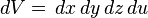$\,dV=\,dx\,dy\,dz\,du$, then: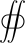${S}$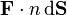$\mathbf{F}\cdot n \, {\rm d}\bold{S}$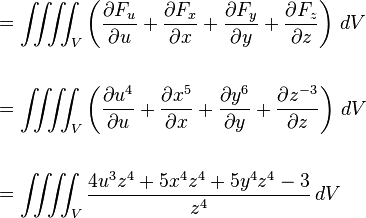\begin{align} &=\iiiint_V \left(\frac{\partial F_u}{\partial u} + \frac{\partial F_x}{\partial x} + \frac{\partial F_y}{\partial y} + \frac{\partial F_z}{\partial z}\right)\,dV\\\\ &=\iiiint_V \left(\frac{\partial u^4}{\partial u} + \frac{\partial x^5}{\partial x} + \frac{\partial y^6}{\partial y} + \frac{\partial z^{-3}}{\partial z}\right)\,dV\\\\ &=\iiiint_V {{\frac{4 u^3 z^4 + 5 x^4 z^4 + 5 y^4 z^4 - 3}{z^4}}}\,dV \end{align}

From this, we now can evaluate the integral: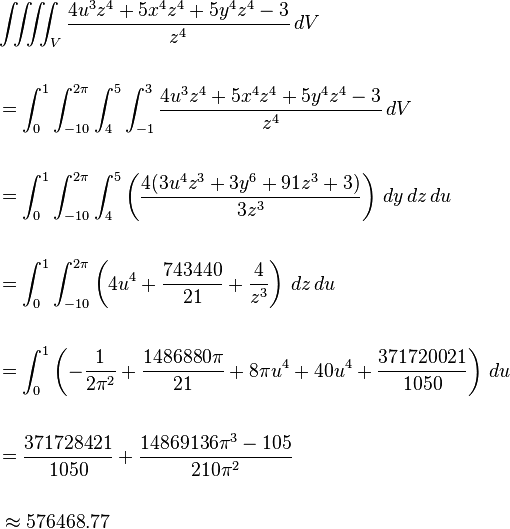\begin{align} &\iiiint_V {{\frac{4 u^3 z^4 + 5 x^4 z^4 + 5 y^4 z^4 - 3}{z^4}}}\,dV\\\\ &=\int_{0}^{1}\int_{-10}^{2\pi}\int_{4}^{5}\int_{-1}^{3} \frac{4 u^3 z^4 + 5 x^4 z^4 + 5 y^4 z^4 - 3}{z^4}\,dV\\\\ &=\int_{0}^{1}\int_{-10}^{2\pi}\int_{4}^{5}\left(\frac{4(3u^4z^3+3y^6+91z^3+3)}{3z^3}\right)\,dy\,dz\,du\\\\ &=\int_{0}^{1}\int_{-10}^{2\pi}\left(4u^4+\frac{743440}{21}+\frac{4}{z^3}\right)\,dz\,du\\\\ &=\int_{0}^{1} \left(-\frac{1}{2\pi^2}+\frac{1486880\pi}{21}+8\pi u^4+40 u^4+\frac{371720021}{1050}\right)\,du\\\\ &=\frac{371728421}{1050}+\frac{14869136\pi^3-105}{210\pi^2}\\\\ &\,\approx{576468.77} \end{align}

Thus, we can evaluate a contour integral of the fourth dimension.

## Images for kidsContour integral Facts for Kids. Kiddle Encyclopedia.